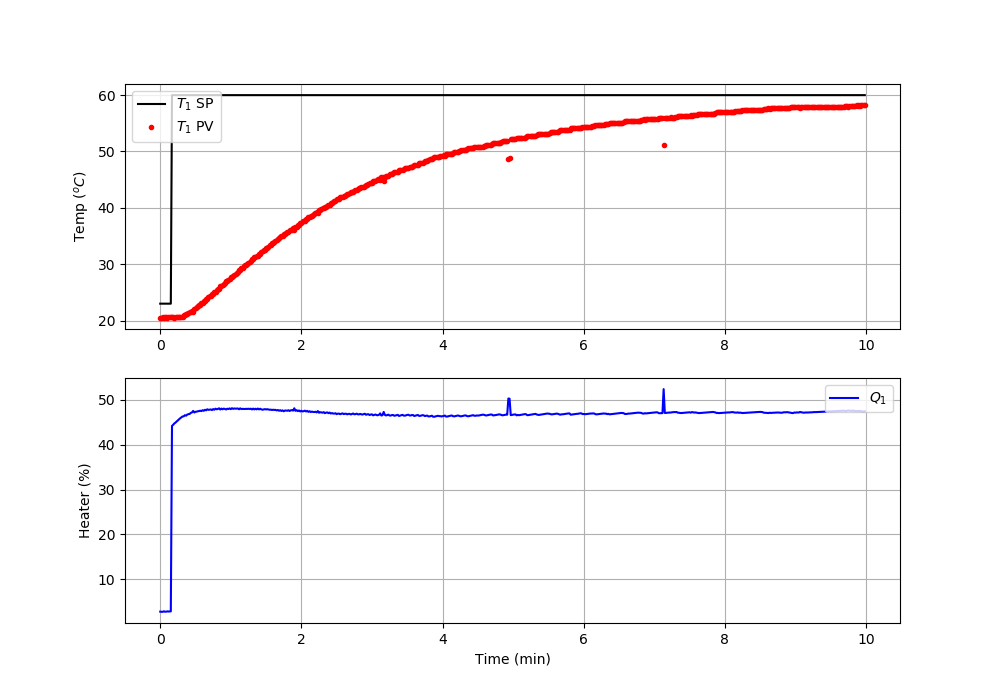Main

## TCLab PI Control

Objective: Implement a discrete PID controller without the derivative term (PI Control). Show the control performance with a setpoint change from 23oC to 60oC.

import numpy as np
import matplotlib.pyplot as plt
import tclab
import time

# -----------------------------
# Input controller gain (Kc)
# Input controller time constant (tauI)
# -----------------------------
Kc =
tauI =

n = 600  # Number of second time points (10 min)
tm = np.linspace(0,n-1,n) # Time values
lab = tclab.TCLab()
T1 = np.zeros(n)
Q1 = np.zeros(n)
# step setpoint from 23.0 to 60.0 degC
SP1 = np.ones(n)*23.0
SP1[10:] = 60.0
Q1_bias = 0.0
for i in range(n):
# record measurement
T1[i] = lab.T1

# --------------------------------------------------
# fill-in PI controller equation to change Q1[i]
# --------------------------------------------------
Q1[i] =

# --------------------------------------------------
# implement new heater value with integral anti-reset windup
Q1[i] = max(0,min(100,Q1[i])) # clip to 0-100%
# --------------------------------------------------

lab.Q1(Q1[i])
if i%20==0:
print(' Heater,   Temp,  Setpoint')
print(f'{Q1[i]:7.2f},{T1[i]:7.2f},{SP1[i]:7.2f}')
# wait for 1 sec
time.sleep(1)
lab.close()
# Save data file
data = np.vstack((tm,Q1,T1,SP1)).T
np.savetxt('PI_control.csv',data,delimiter=',',\

# Create Figure
plt.figure(figsize=(10,7))
ax = plt.subplot(2,1,1)
ax.grid()
plt.plot(tm/60.0,SP1,'k-',label=r'$T_1$ SP')
plt.plot(tm/60.0,T1,'r.',label=r'$T_1$ PV')
plt.ylabel(r'Temp ($^oC$)')
plt.legend(loc=2)
ax = plt.subplot(2,1,2)
ax.grid()
plt.plot(tm/60.0,Q1,'b-',label=r'$Q_1$')
plt.ylabel(r'Heater (%)')
plt.xlabel('Time (min)')
plt.legend(loc=1)
plt.savefig('PI_Control.png')
plt.show()

A proportional integral (PI) controller is a variation of the PID controller without the derivative term. The controller is an equation that adjusts the controller output, u(t), for input into the system as the manipulated variable. It is a calculation of the difference between the setpoint SP and process variable PV. The adjustable parameters are the controller gain, K_c, and controller reset time or integral time constant, \tau_I. A large gain or small integral time constant produces a controller that reacts aggressively to a difference between the measured PV and target SP.

$$Q(t) = Q_{bias} + K_c \, \left( T_{SP}-T_{PV} \right) + \frac{K_c}{\tau_I}\int_0^t \left( T_{SP}-T_{PV} \right) dt$$

$$\quad = Q_{bias} + K_c \, e(t) + \frac{K_c}{\tau_I}\int_0^t e(t) dt$$

The Q_{bias} term is a constant that is typically set to the value of Q(t) when the controller is first switched from manual to automatic mode. The deviation variable for the heater is the change in value Q'(t) = Q(t) - Q_{bias}. For the TCLab, Q_{bias}=0 because the TCLab starts with the heater off. The Q_{bias} gives bumpless transfer if the error is zero when the controller is turned on. The error from the set point is the difference between the T_{SP} and T_{PV} and is defined as e(t) = T_{SP} - T_{PV}. The continuous integral is approximated in a discrete form as a summation of the error multiplied by the sample time.

$$\frac{K_c}{\tau_I}\int_0^t e(t) dt \approx \frac{K_c}{\tau_I} \sum_{i=1}^{n_t} e_i(t)\Delta t$$

See additional information on PI controllers. Use a basic tuning correlation with FOPDT parameters (K_c and \tau_p) determined from the TCLab graphical fitting or TCLab regression exercises.

$$K_c = \frac{1}{K_p} \quad \quad \tau_I = \tau_p$$

Fill in the values of K_c, \tau_I, and the PI equation in the code below. Add anti-reset windup to prevent the integral from accumulating when the heater is saturated at 0% or 100%.

Solutionimport numpy as np
import matplotlib.pyplot as plt
import tclab
import time

# -----------------------------
# Input controller gain (Kc)
# Input controller time constant (tauI)
# -----------------------------
Kc = 1/0.9
tauI = 175.0

n = 600  # Number of second time points (10 min)
tm = np.linspace(0,n-1,n) # Time values
lab = tclab.TCLab()
T1 = np.zeros(n)
Q1 = np.zeros(n)
# step setpoint from 23.0 to 60.0 degC
SP1 = np.ones(n)*23.0
SP1[10:] = 60.0
Q1_bias = 0.0
ierr = 0.0
for i in range(n):
# record measurement
T1[i] = lab.T1

# --------------------------------------------------
# fill-in PI controller equation to change Q1[i]
# --------------------------------------------------
err = SP1[i]-T1[i]
ierr += err
Q1[i] = Q1_bias + Kc*err + Kc/tauI * ierr

# --------------------------------------------------
# implement new heater value with integral anti-reset windup
if Q1[i]>=100:
Q1[i]=100
ierr -= err
if Q1[i]<=0:
Q1[i]=0
ierr -= err
# --------------------------------------------------

lab.Q1(Q1[i])
if i%20==0:
print(' Heater,   Temp,  Setpoint')
print(f'{Q1[i]:7.2f},{T1[i]:7.2f},{SP1[i]:7.2f}')
# wait for 1 sec
time.sleep(1)
lab.close()
# Save data file
data = np.vstack((tm,Q1,T1,SP1)).T
np.savetxt('PI_control.csv',data,delimiter=',',\

# Create Figure
plt.figure(figsize=(10,7))
ax = plt.subplot(2,1,1)
ax.grid()
plt.plot(tm/60.0,SP1,'k-',label=r'$T_1$ SP')
plt.plot(tm/60.0,T1,'r.',label=r'$T_1$ PV')
plt.ylabel(r'Temp ($^oC$)')
plt.legend(loc=2)
ax = plt.subplot(2,1,2)
ax.grid()
plt.plot(tm/60.0,Q1,'b-',label=r'$Q_1$')
plt.ylabel(r'Heater (%)')
plt.xlabel('Time (min)')
plt.legend(loc=1)
plt.savefig('PI_Control.png')
plt.show()